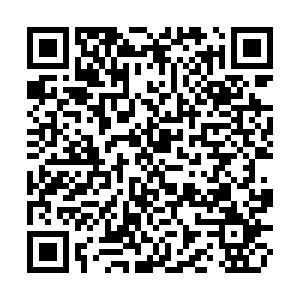## 留言板引用本文: 陈辉, 曾文爱, 连峰, 韩崇昭. 水平集高斯过程的非星凸形扩展目标跟踪算法[J]. 电子与信息学报.CHEN Hui, ZENG Wenai, LIAN Feng, HAN Chongzhao. Non-Star-Convex Extended Target Tracking Algorithm for Level-Set Gaussian Process[J]. Journal of Electronics & Information Technology. doi: 10.11999/JEIT220997
 Citation: CHEN Hui, ZENG Wenai, LIAN Feng, HAN Chongzhao. Non-Star-Convex Extended Target Tracking Algorithm for Level-Set Gaussian Process[J]. Journal of Electronics & Information Technology.• 中图分类号: TP274

## Non-Star-Convex Extended Target Tracking Algorithm for Level-Set Gaussian Process

Funds: The National Natural Science Foundation of China (61873116, 62163023), The Industrial Support Project of Education Department of Gansu Province (2021CYZC-02), The Science and Technology Program of Gansu Province (20JR10RA184)
• 摘要: 针对复杂环境下的非星凸形不规则形状扩展目标跟踪问题，该文提出基于能量泛函的水平集高斯过程扩展目标跟踪算法。首先，利用水平集随机超曲面模型(Level-Set RHM)通过多边形方法对形状内部进行建模。然后，用高斯过程(GP)学习Level-Set建模输入与输出的非线性映射关系，以求得边界函数最大值，并进一步推导Level-Set与GP相融合的非线性量测方程。在最优非线性滤波的框架下，最终推导得到水平集高斯过程(Level-Set GP)算法，并利用面积差作为不规则形状扩展目标形状估计的评价指标。仿真实验表明了所提算法对非星凸形不规则形状扩展目标形状估计的有效性。
• 图  1  不规则扩展目标形状

图  2  非星凸形扩展目标跟踪

图  3  求边界形状函数最大值过程

图  4  不同形状的跟踪效果

图  5  不同形状的面积误差

图  6  不同噪声下的面积误差

图  7  动态目标跟踪

图  8  不同时刻动态目标的面积误差

图  9  群目标跟踪

图  10  群目标面积误差

 算法1 Level-Set GP模型算法部分伪码 输入：${\boldsymbol{x} }_{ { {k} } - 1|{ {k} } - 1}^{ {\rm{f} } } , { {\boldsymbol{F} }^{\rm{f} } }, {{\boldsymbol{Q}}_{ {k} } }, {\boldsymbol{x}}_0^{{\rm{p}}} , {{\boldsymbol{P}}_{ { {k} } - 1|{ {k} } - 1} }, {{\boldsymbol{C}}_{ { {k} } - 1|{ {k} } - 1} }, {\boldsymbol{F}}_{ {k} }^{{\rm{p}}} , {\boldsymbol{Q}}_{ {k} }^{{\rm{p}}}$ 步骤1 预测 for${{ }}k = 1{{ : }}N$ ${\boldsymbol{x} }_{ { {k} }|{ {k} } - 1}^{\rm{ {f} } } = { {\boldsymbol{F} }^{ {\rm{f} } } }{\boldsymbol{x} }_{ { {k} } - 1|{ {k} } - 1}^{{\rm{f}}}$; ${{\boldsymbol{P}}_{ { {k} }|{ {k} } - 1} } = {{\boldsymbol{F}}^{\rm{f}}}{{\boldsymbol{P}}_{ { {k} } - 1|{ {k} } - 1} }{({{\boldsymbol{F}}^{\rm{f}}})^{{\rm{T}}} } + {{\boldsymbol{Q}}_{ {k} } }$; 根据式(10)—式(11)，计算得到${{k}}$时刻扩展形状参数的预测值; ${{\boldsymbol{C}}_{ { {k} }|{ {k} } - 1} } = {\boldsymbol{F}}_{ {k} }^{{\rm{p}}} {{\boldsymbol{C}}_{ { {k} } - 1|{ {k} } - 1} }{({\boldsymbol{F}}_{ {k} }^{{\rm{p}}} )^{{\rm{T}}} } + {\boldsymbol{Q}}_{ {k} }^{{\rm{p}}}$; end for 步骤2 更新 for $k = 1:N$ for ${{ }}j = 1:{\rm{num}}$ 根据式(43)计算$k$时刻边界形状函数最大值; 计算伪量测方程传递后的样本点$h_{k,j}^*$; 根据式(47)—式(52)，更新扩展形状参数${\boldsymbol{x}}_{ {k} }^{{\rm{p}}}$和协方差${{\boldsymbol{C}}_{ { {k} }|{ {k} } } }$; end for end for 输出：${\boldsymbol{x}}_{ {k} }^{{\rm{p}}} , {{\boldsymbol{C}}_{ { {k} }|{ {k} } } }$
 算法2　面积差算法 输入：${{x,}}{{y,}}{{X,}}{{Y}}$ ${ {\rm{poly} } } 1 = { {\rm{polyshape} } } ({{x} },{{y} })$; ${{\rm{poly}}} 2 = {{\rm{polyshape}}} ({{X} },{{Y} })$; ${{\rm{intpoly}}} = {{\rm{intersect}}} ({{\rm{poly}}} 1,{{\rm{poly}}} 2)$; ${{\rm{intarea}}} = {{\rm{polyarea}}} ({{\rm{intpoly}}} (:,1),{{\rm{intpoly}}} (:,2))$; ${{\rm{unipoly}}} = { {\rm{union} } } ({ {\rm{poly} } } 1,{ {\rm{poly} } } 2)$; ${{\rm{uniarea}}} = {{\rm{polyarea}}} ({{\rm{unipoly}}} (:,1),{{\rm{unipoly}}} (:,2))$; ${ {\rm{trueshape} } } = { {\rm{polyarea} } } ({{X,Y} })$; 根据式(54), 计算面积误差$\epsilon$。 输出：$\epsilon$
•点击查看大图
##### 计量
• 文章访问数:  258
• HTML全文浏览量:  118
• PDF下载量:  50
• 被引次数: 0
##### 出版历程
• 收稿日期:  2023-01-11
• 录用日期:  2023-01-11
• 网络出版日期:  2023-01-14

### 目录/下载:  全尺寸图片 幻灯片
• 分享
• 用微信扫码二维码

分享至好友和朋友圈